# Let two non-collinear unit vectors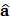and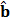from and acute angle. A pointmoves so that at any timethe position vector(where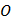is the origin) is given by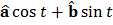. Whenis farthest form origin, let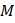be the length ofand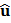be the unit vector along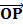Then, a)b)c)d)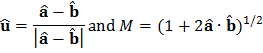## Question ID - 54030 :- Let two non-collinear unit vectorsandfrom and acute angle. A pointmoves so that at any timethe position vector(whereis the origin) is given by. Whenis farthest form origin, letbe the length ofandbe the unit vector alongThen, a)b)c)d)3537

(a)

Given,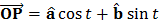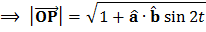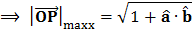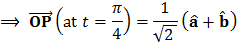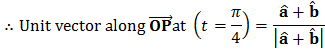Next Question :
 The ratio between a two-digit number and the sum of the digits of that number is 4:1. If the digit in the unit’s place is 3 more than the digit in the ten’s place, then the number is (a) 24 (b) 36 (c) 63 (d) 96